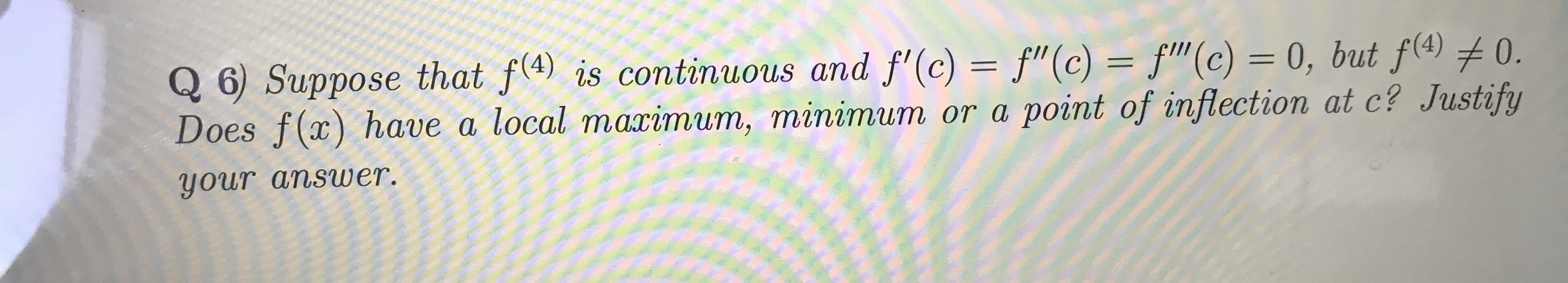# Q 6) Suppose that f4) is continuous and f'(c)Does f(x) have a local maximum, minimum or a point of inflection at c? Justify= f"(c) = f"(c) = 0, but f(4)0.your answer.

Question
12 viewshelp_outlineImage TranscriptioncloseQ 6) Suppose that f4) is continuous and f'(c) Does f(x) have a local maximum, minimum or a point of inflection at c? Justify = f"(c) = f"(c) = 0, but f(4)0. your answer. fullscreen
check_circle

Step 1

At x=c both f'(c) and f"(c) equals 0. This means we can not have any local minimum or maximum at x=c.

Because f"(c)=0 means f'(x) is neither...

### Want to see the full answer?

See Solution

#### Want to see this answer and more?

Solutions are written by subject experts who are available 24/7. Questions are typically answered within 1 hour.*

See Solution
*Response times may vary by subject and question.
Tagged in

### Calculus